# Introduction to TensorFlow

TensorFlow is an open-source software library designed by the Google team to facilitate machine learning and deep learning concepts in the most straightforward manner. It is a comprehensive framework that manages all aspects of a machine learning system through its highly flexible architecture that leverages different processing units like CPU, GPU, or TPU to execute computations. It provides a collection of workflows to develop and train models using Python, Swift, or Javascript and effortlessly deploy in the cloud, in the browser, or on-device irrespective of the language you use.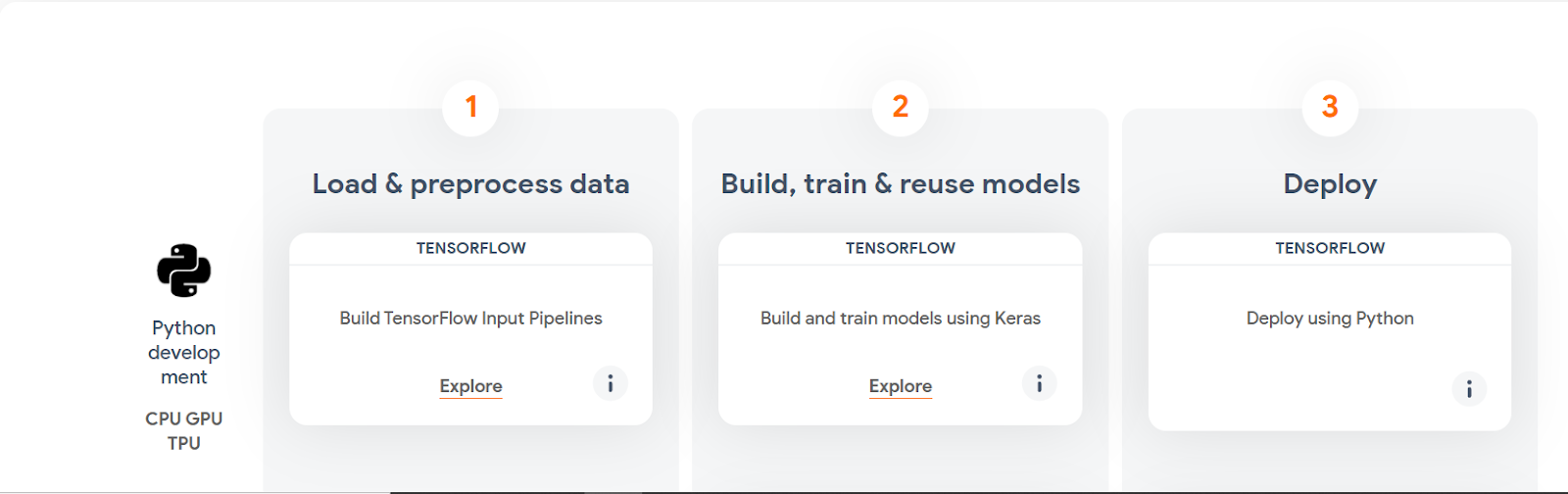Source: Tensorflow overview

A Tensorflow program can be divided into two significant steps. It first builds a computational graph, a graph of all the operations to be done arranged into a graph of nodes. The next step is to evaluate the graph for running within a session that encapsulates the TensorFlow runtime’s control and state. Tensorflow is highly scalable as it manipulates internal data representation to tensors, which are multi-dimensional arrays.

Creating a computational graph refers to the process of defining the nodes. Tensorflow provides a variety of nodes for various tasks. A node can take zero or more tensors as inputs and produce a tensor as an output. In order to run the computational graph, we need to create a session that can be created using the following command:

``sess = tf. Session()``

Here is a sample Tensorflow program to explain the working:

``` # importing tensorflow
import tensorflow.compat.v1 as tf
# creating a computational graph using nodes
with tf.get_default_graph().as_default():
node1 = tf.constant(12, dtype=tf.int32)
node2 = tf.constant(5, dtype=tf.int32)
# creating a tensorflow session
sess = tf.Session()
# evaluating node3 and printing the result
print("The resulting sum is:",sess.run(node3))
# closing the session
sess.close()
```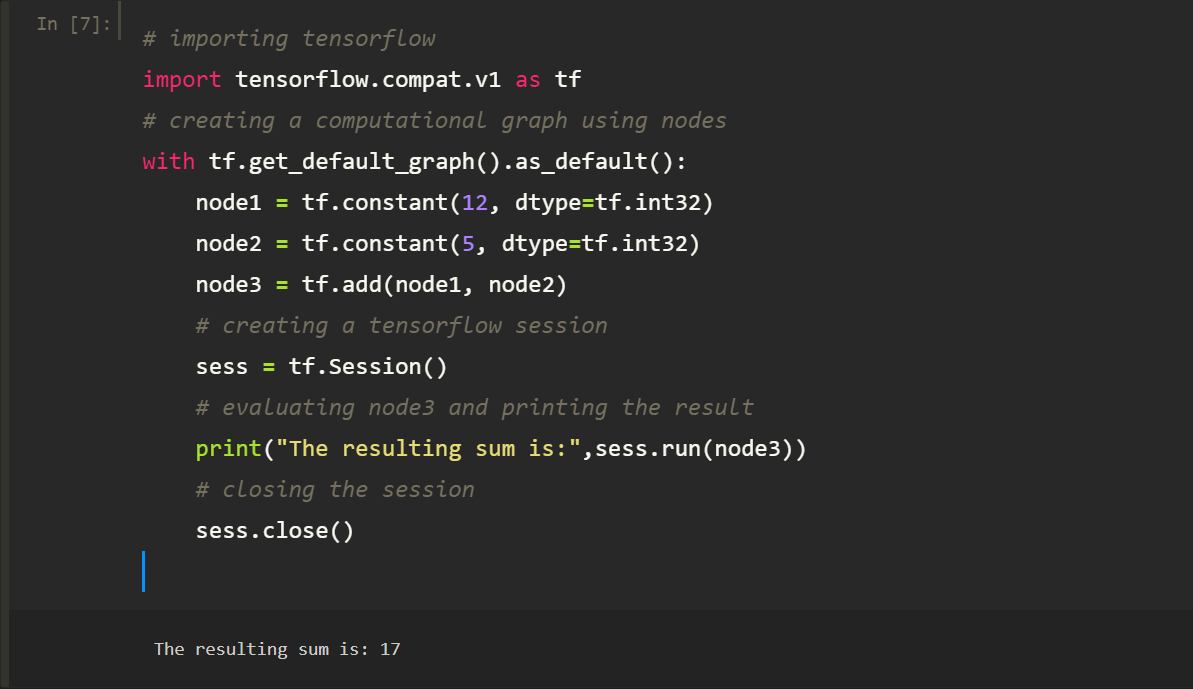TensorFlow provides multiple APIs, which can broadly be categorized into low-level APIs and high-level APIs.Machine learning researchers use the low-level APIs to provide better control over the models that cater to them to create and explore new machine learning algorithms. APIs are arranged hierarchically such that the high-level APIs are built on the low-level APIs. High-level APIs are easier to use and learn than low-level APIs and make repetitive tasks easier to implement. Keras is one such high-level API that runs on top of Tensorflow and helps build network layers quickly.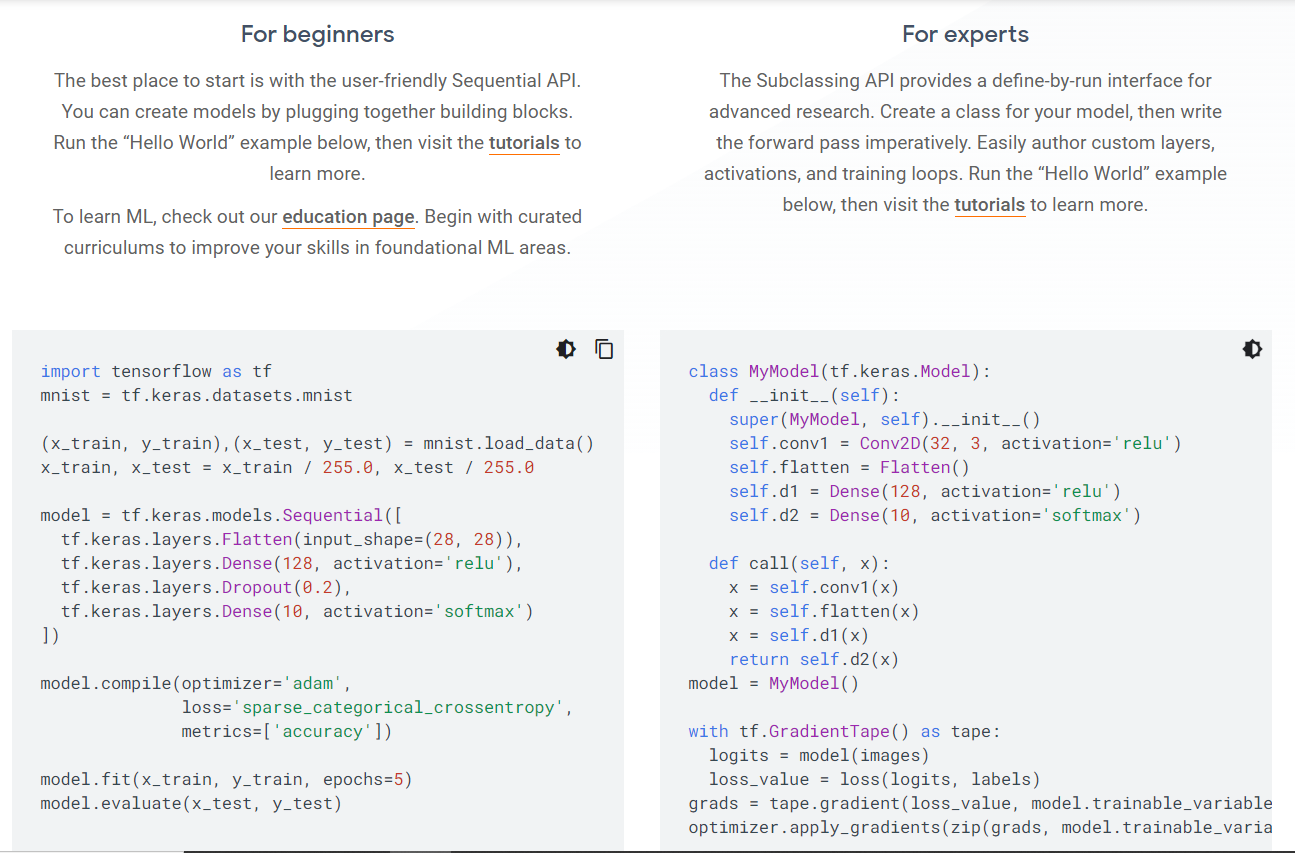Source:Tensorflow overview

We will use Keras API on top of Tensorflow to build a neural network that works as an image classifier. The dataset we will use is the MNIST dataset, which contains thousands of images of handwritten digits.

Write the following code to build your first neural network:

``````# import Tensorflow
import tensorflow as tf
mnist = tf.keras.datasets.mnist
(x_train, y_train), (x_test, y_test) = mnist.load_data()
x_train, x_test = x_train / 255.0, x_test / 255.0
# Create The Neural network layers using the Keras sequential model
model = tf.keras.models.Sequential([
tf.keras.layers.Flatten(input_shape=(28, 28)),
tf.keras.layers.Dense(128, activation='relu'),
tf.keras.layers.Dropout(0.2),
tf.keras.layers.Dense(10)
])
# The model returns a vector of scores for each possible class, softmax converts it into probabilities
predictions = model(x_train[:1]).numpy()
tf.nn.softmax(predictions).numpy()
# The losses function returns a scalar loss corresponding to each example
loss_fn = tf.keras.losses.SparseCategoricalCrossentropy(from_logits=True)
loss_fn(y_train[:1], predictions).numpy()
# The compile function compiles the model on the basis of the given optimizer and metrics
loss=loss_fn,
metrics=['accuracy'])
# The fit function adjusts the parameters to minimize the loss
model.fit(x_train, y_train, epochs=5)
# The evaluate function evaluates the model on the basis of test-set or validation-set
model.evaluate(x_test, y_test, verbose=2)
``````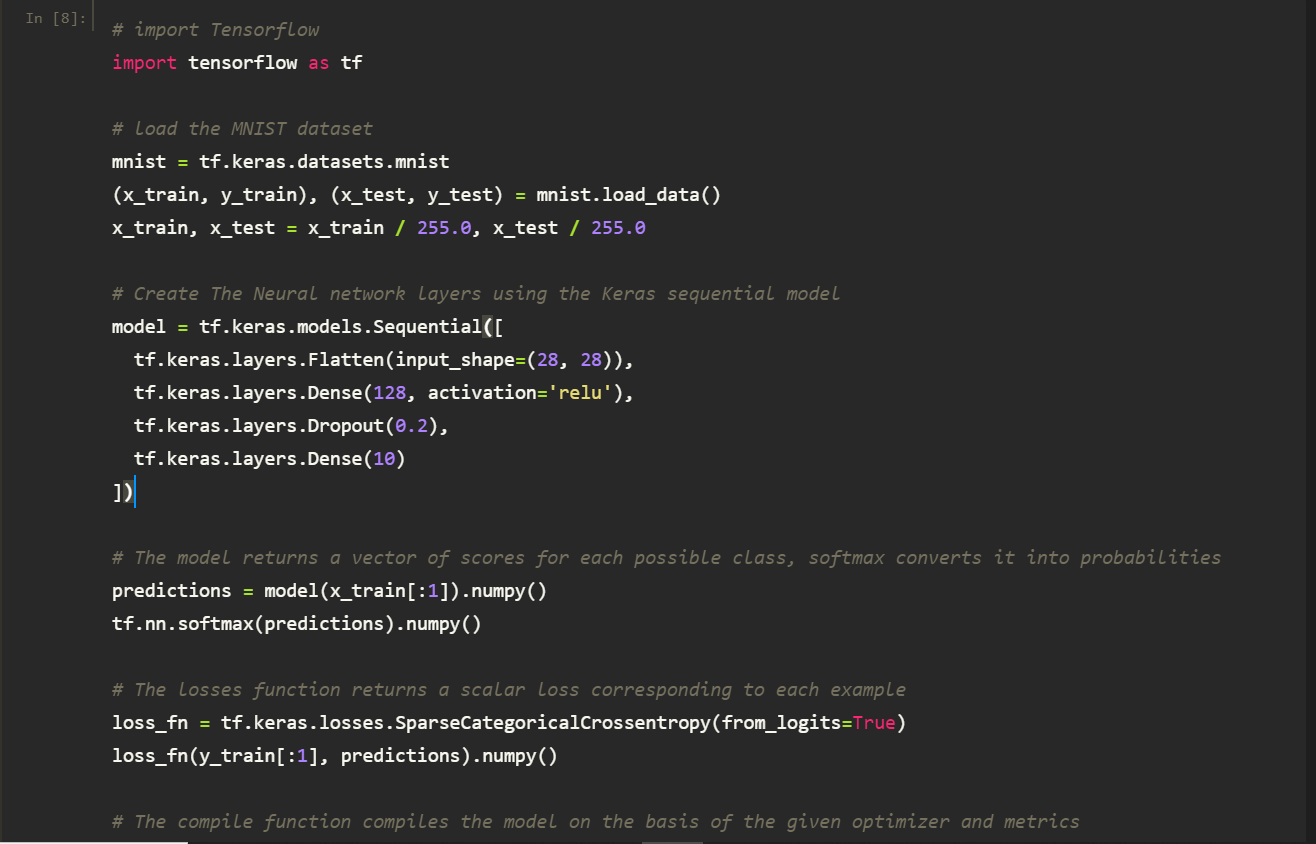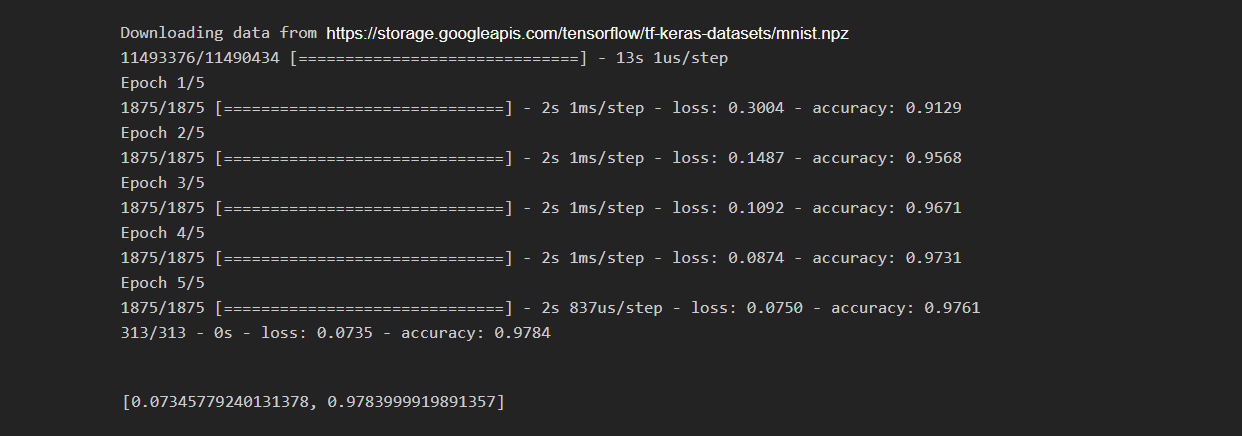Our model is trained at an accuracy of approximately 98% !!

Check out TensorFlow official repository to learn about Tensorflow in depth and continue your journey into the field of deep learning!!.

##### Nitish Kumar

Nitish is a computer science undergraduate with keen interest in the field of deep learning. He has done various projects related to deep learning and closely follows the new advancements taking place in the field.